• 一维到多维理解卡尔曼滤波 写在前面 本文以移动机器人定位问题(Localization)为例 借鉴Udacity课程：AI for Robotics 对于一切问题，降维思考都是个好办法 卡尔曼滤波的核心思想 根据上一个状态得到当前状态的 ...
从一维到多维理解卡尔曼滤波
写在前面

本文以移动机器人定位问题(Localization)为例
借鉴Udacity课程：AI for Robotics
对于一切问题，降维思考都是个好办法

卡尔曼滤波的核心思想

根据上一个状态得到当前状态的 “带误差的预测值”；
在当前状态使用某种测量工具得到 “带误差的测量值”；
根据上述两个值计算得到当前状态的最优值；
在当前状态的最优值基础上，加上该状态要发生的动作，得到下一状态带误差的预测值

一维卡尔曼滤波
一维高斯分布 (Gaussian Distribution)
要讲卡尔曼滤波，就不得不说高斯分布，因为**“带误差的预测值”和“带误差的测量值”均为高斯分布**，卡尔曼滤波的工作就是整合这两个值的高斯分布。
任意高斯分布都可以由 $(\mu , \sigma^2)$来表征，$\mu$ 为高斯分布的均值，$\sigma^2$则为方差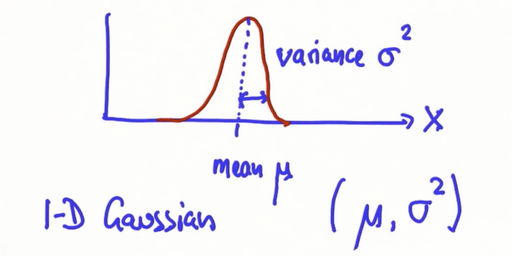由图可明显看出，$(\mu , \sigma^2)$大小对高斯分布的影响如下：

$\mu$决定了高斯分布对称轴或者说峰值的位置
$\sigma^2$决定了高斯分布的宽度（和高度）。

$\sigma^2$越大，误差越大，Gaussian越矮胖；
反之，误差越小，Gaussian越高瘦；
由于Gaussian曲线下的面积恒等于1，就决定了越宽的Gaussian峰值越小，越窄的Gaussian峰值越高。

公式
这里有必要给出Gaussian的公式：
$f(x)=\frac{1}{\sqrt{2 \pi \sigma^{2}}} \exp \left[\frac{-1}{2} \frac{(x-\mu)^{2}}{\sigma^{2}}\right]$
卡尔曼滤波利用Gaussian在重复地做这两件事：

上述两个值的更新方式分两种：直接乘积(product) 和 卷积(convolution)

对于测量值使用直接乘积，贝叶斯定理来计算
对于预测值使用卷积，

现假设你正在定位一个机器人，位置的先验分布（上一步的预测值，在下一节介绍）为黑色曲线，接着由传感器测量得到了如蓝色曲线一样的测量值曲线。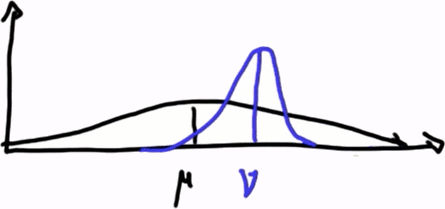那么很明显两个数据融合之后的Gaussian变为

均值在 $\mu$ 和 $\nu$之间，且更靠近 $\nu$（因为蓝色曲线的方差更小，对位置的估计更加精确，所以我们更信任它），
峰值也较两个组成的Gaussian更高（因为在借鉴了两组数据之后，我们对位置更加确信，方差更小）

公式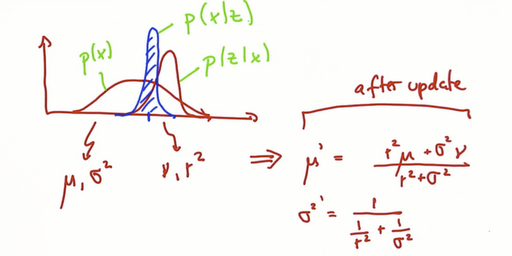测量值Gaussian参数按如下公式更新
$\mu^{\prime}=\frac{r^{2} \mu+\sigma^{2} \nu}{r^{2}+\sigma^{2}}$
${\sigma^{2}}^{\prime}=\frac{1}{\frac{1}{r^{2}}+\frac{1}{\sigma^{2}}}$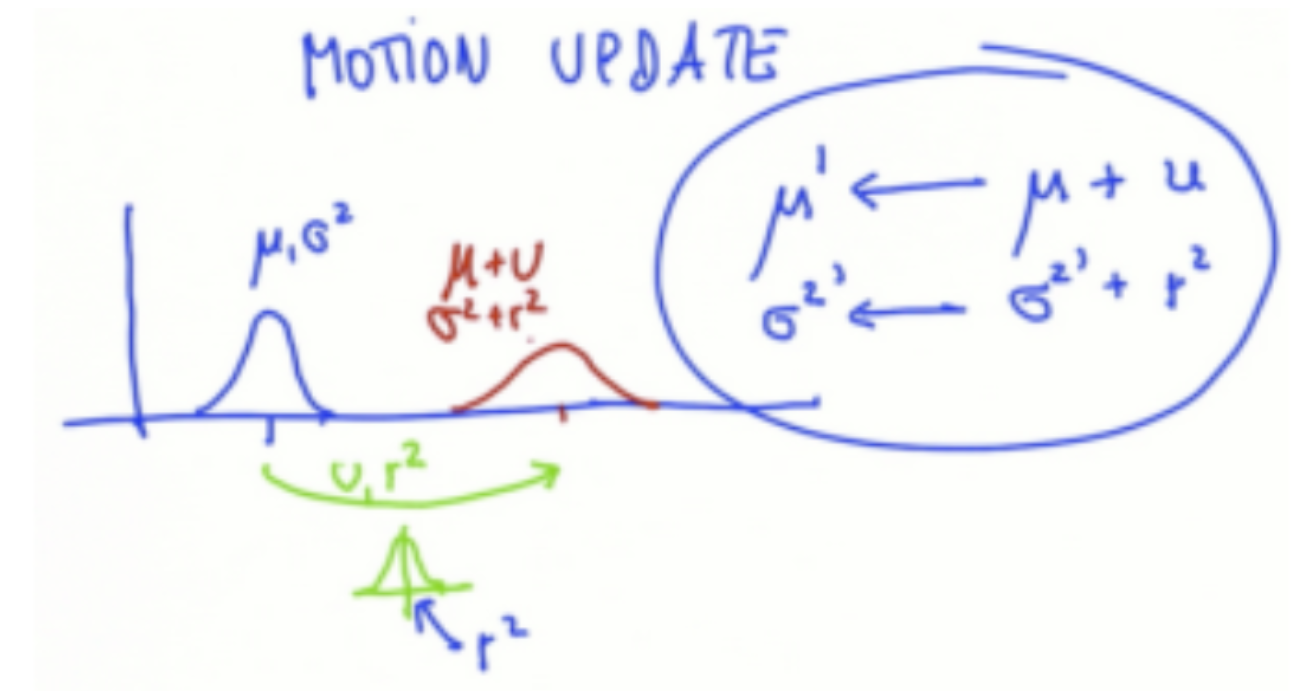预测值的更新就简单了，均值和方差都是直接求和。当我们将预测值视作机器人的动作更新的话，就好理解了。蓝色曲线代表目前位置的最优估计（根据上一节的测量值更新得到），绿色曲线代表下一步要运动的距离（有误差，可以理解为要让电机转多少圈）。那么下一时刻的预测位置是不是就是目前位置+运动距离了？误差是不是也变成了二者误差的和了？
预测值Gaussian参数按如下公式更新：
$\begin{array}{l}{\mu^{\prime} = \mu+\nu} \\ {{\sigma^{2}}^{\prime} = \sigma^{2}+r^{2}}\end{array}$
总结
以上即为一维卡尔曼滤波。总结一下：

我们使用测量值更新来融合 传感器测量到的带误差的位置信息 以及 基于上一状态的预测信息，得到当前状态的最优位置估计 (Measurement updates)
根据 下一步要进行的动作 以及 当前状态的最优位置估计，得到下一状态的预测值 (Prediction/Motion updates)

卡尔曼滤波就是这么简单的两步循环，不断借助多方数据，逼近实际值。
多维卡尔曼滤波
在多维空间中，卡尔曼滤波不仅能估计位置（传感器仅能够测量位置），还能够根据数据估计出速度信息，因此多为卡尔曼滤波可以综合速度信息估计出未来位置。
多元高斯
设空间维度为n

均值是一个n维的向量
方差变为协方差，是一个 $n \times n$ 阶矩阵

以二维高斯为例， $(x_0,y_0)$表示均值，两个维度之间是相关的。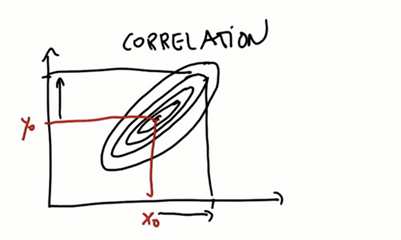等高线越小的Gaussian不确定性越小，下图横轴上的不确定性很小，但纵轴方向的不确定性很大。如果将横轴视作位置，纵轴视作速度，我们根据单次测量值得到的Gaussian就是这样。（因为我们假设传感器只能测量到位置信息，所以速度的不确定性巨大）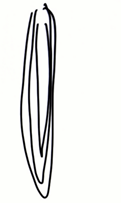以(1,0)为起点，速度为1，则下一时刻应在(2,1)点
同理，以(1,0)为起点，速度为2，则下一时刻应在(3,2)点
由此可知蓝色的一维信息和红色的二维信息是有关系的。
测量值更新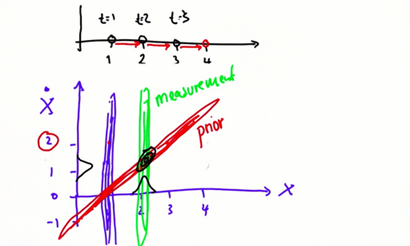接下来，单看第二状态的测量值（绿色等高线），将其与状态先验（红色等高线）相乘，即得到该状态速度和位置的最优估计（黑色等高线），很明显得到的估计值只有很小的不确定性。
预测值更新
新位置的预测值只有位置信息，和一维的情况一样，公式为
$x^{\prime}=x+u$
新位置也可以视作当前位置加上速度
多维卡尔曼滤波器设计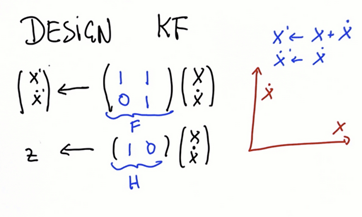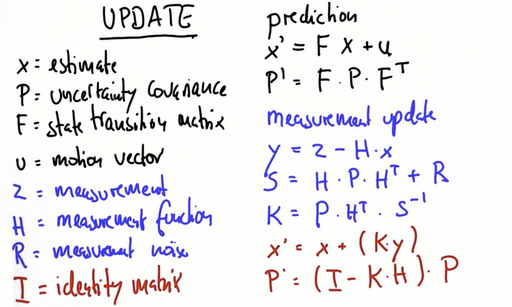参数说明
预测值更新公式

$x$: 当前位置估计
$x'=Fx+u$

$P$: 协方差矩阵
$P'=FPF^T$

$F:$ 状态转移矩阵
测量值更新公式

$u$: 动作向量
$y=Z-Hx$

$Z:$ 测量值
$S=HPH^T+R$

$H:$ 测量矩阵
$K=PH^TS^{-1}$

$R:$ 测量误差
$x'=x+Ky$

$I:$ 单位矩阵
$P'=(I-KH)P$


展开全文Kalman
• 数组前言一、一维数组1、一维数组的创建2、一维数组的使用2.1数组表示法2.2指针表示法3、一维数组在内存上的映射二、多维数组1、多维数组的创建2、多维数组的使用2.1数组表示法2.2指针表示法3、二维数组在内存上的...
数组前言一、一维数组1、一维数组的创建2、一维数组的使用2.1数组表示法2.2指针表示法3、一维数组在内存上的映射二、多维数组1、多维数组的创建2、多维数组的使用2.1数组表示法2.2指针表示法3、二维数组在内存上的映射三、数组的实战运用1、用一维数组存储整数1到100并求和2、图形打印
前言
数组（Array）是一种数组结构，它把具有相同的数据类型的变量存储到一块内存单元地址连续的内存空间中。通常我们又把数组里面的变量称为元素。
一、一维数组
1、一维数组的创建
1.1在栈区中创建
//方式一：显式声明法
int array={1,2,3,4};//声明并定义一个含有4个整型元素的整型数组
//方式二：隐式声明法
int array[]={1,2,3,4};//同上

1.2在堆区中创建
//在堆区开辟一个含有4个整型内存空间，并用一个整型指针指向该空间
int *array=(int *)malloc(sizeof(int)*4);

2、一维数组的使用
2.1数组表示法
2.1.1数组元素的获取
int array={1,2,3,4};
//采用下标索引
printf("array=%d\n",array);
printf("array=%d\n",array);
printf("array=%d\n",array);
printf("array=%d\n",array);
//采用遍历的方式
for(int i=0;i<4;i++)
{
printf("array[%d]=%d\n",i,array[i]);
}

2.1.2数组的函数传值
void print_array(int array)
{
for(int i=0;i<4;i++)
{
printf("array[%d]=%d\n",i,array[i]);
}
}
int main()
{
int array={1,2,3,4};
print_array(array);
return 0;
}

2.2指针表示法
2.2.1数组元素的获取
int array={1,2,3,4};
printf("a=%d\n",*(array+0));//在编译过程中*(array+0)将转换成*(array+0*sizeof(int))
printf("a=%d\n",*(array+1));//在编译过程中*(array+0)将转换成*(array+1*sizeof(int))
printf("a=%d\n",*(array+2));//在编译过程中*(array+0)将转换成*(array+2*sizeof(int))
printf("a=%d\n",*(array+3));//在编译过程中*(array+0)将转换成*(array+3*sizeof(int))
//遍历
for(int i=0;i<4;i++)
{
printf("a[%d]=%d\n",i,*(array+i));
}

2.2.2数组的函数传值
void print_array(int *array)
{
for(int i=0;i<4;i++)
{
printf("a[%d]=%d\n",i,array[i]);
}
}
int main()
{
int array={1,2,3,4};
print_array(array);
return 0;
}

3、一维数组在内存上的映射代码验证如下
	int array={1,2,3,4};
printf("array的地址为：%p\n",&array);
printf("array的地址为：%p\n",&array);
printf("array的地址为：%p\n",&array);
printf("array的地址为：%p\n",&array);


VC6.0运行结果如下二、多维数组
对于一维数组，我们可以这样理解，一个小号水果篮子里面放着n个水果，而多维
数组，以二维数组为例，可以理解为——n个小号水果篮子,每个水果篮子里面放着n
个水果，而以三维数组为例，可以理解为——n个中号水果篮子，每个中号水果篮
子里面放着n个小号水果篮子，而每个小号水果篮子里面又放着n个水果...可以这么
说，n维数组套n层娃（俄罗斯套娃哈哈哈）

1、多维数组的创建
1.1在栈区中创建
int array={{1,2},{3,4}};//创建一个二维数组
int array={{{1,2},{3,4}},		//创建一个三维数组
{{5,6},{7,8}}
};

在堆区中创建
//以二维数组为例
int **array=(int **)malloc(2*sizeof(int *));
for(int i=0;i<2;i++)
{
array[i]=(int *)malloc(2*sizeof(int));
}

2、多维数组的使用
2.1数组表示法
2.1.1数组元素的获取
//以二维数组为例
//采用下标索引
int array={{1,2},{3,4}};
printf("array=%d\n",array);
printf("array=%d\n",array);
printf("array=%d\n",array);
printf("array=%d\n",array);
//采用遍历的方式
for(int i=0;i<2;i++)
{
for(int j=0;j<2;j++)
{
printf("array[%d][%d]=%d\n",i,j,array[i][j]);
}
}


2.1.2数组的函数传值
void print_array(int array)//也可以写成int array[],但是不能写成int array[]
{
for(int i=0;i<2;i++)
{
for(int j=0;j<2;j++)
{
printf("a[%d][%d]=%d\n",i,j,array[i][j]);
}
}
}
int main()
{
int array={{1,2},{3,4}};
print_array(array);
return 0;
}

2.2指针表示法
2.1.1数组元素的获取
int **array=(int **)malloc(2*sizeof(int *));
for(int i=0;i<2;i++)
{
array[i]=(int *)malloc(2*sizeof(int));
}
int num=1
for(int i=0;i<2;i++)
{
for(int j=0;j<2;j++)
{
array[i][j]=num++;
}
}
for(int i=0;i<2;i++)
{
for(int j=0;j<2;j++)
{
printf("a[%d][%d]=%d\n",i,j,array[i][j]);
}
}

2.1.2数组的函数传值
void print_array(int (*array))
{
for(int i=0;i<2;i++)
{
for(int j=0;j<2;j++)
{
printf("a[%d][%d]=%d\n",i,j,array[i][j]);
}
}
}
int main()
{
int array={{1,2},{3,4}};
print_array(array);
return 0;
}

3、二维数组在内存上的映射
首先我们先看看二维数组的逻辑结构，如下但是其实多维数组在底层的内存存储和一维数组是一样的代码验证如下
	int array={{1,2},{3,4}};
printf("array的地址：%p\n",&array);
printf("array的地址：%p\n",&array);
printf("array的地址：%p\n",&array);
printf("array的地址：%p\n",&array);

VC6.0运行结果如下三、数组的实战运用
编译器——VC6.0
1、用一维数组存储整数1到100并求和
int a={0};//初始化为0
int sum=0;
for(int i=0;i<100;i++)//赋值
{
a[i]=i+1;
}
for(i=0;i<100;i++)		//求和
{
sum+=a[i];
}
printf("从1加到100的和为：%d\n",sum);

2、图形打印
int a={{1},{2},{3},{4}};
for(int i=0;i<4;i++)
{
for(int j=1;j<=a[i];j++)
{
printf("*");
}
printf("\n");
}

运行结果如下int a={{1},{2},{3},{4}};
for(int i=0;i<4;i++)
{
for(int k=5;k>a[i];k--)
{
printf("*");
}

printf("\n");

}

运行结果如下展开全文c语言
• 二、一维数据可视化 三、二维数据可视化 四、多维数据可视化 五、参考资料 一、iris数据集介绍 iris数据集有150个观测值和5个变量，分别是sepal length、sepal width、petal length、petal width、species，其中...
目录

一、iris数据集介绍
二、一维数据可视化
三、二维数据可视化
四、多维数据可视化
五、参考资料

一、iris数据集介绍

iris数据集有150个观测值和5个变量，分别是sepal length、sepal width、petal length、petal width、species，其中species有3个取值：setosa、virginica、versicolor，反正就是鸾尾花的3个不同品种吧，各有50个观测值。具体见下表。

import numpy as np
import pandas as pd
import matplotlib.pyplot as plt
import seaborn as sns

%matplotlib inline
sns.set(style="white", color_codes=True)

#加载iris数据集
iris = pd.DataFrame(iris_data['data'], columns=iris_data['feature_names'])
iris = pd.merge(iris, pd.DataFrame(iris_data['target'], columns=['species']), left_index=True, right_index=True)
labels = dict(zip([0,1,2], iris_data['target_names']))
iris['species'] = iris['species'].apply(lambda x: labels[x])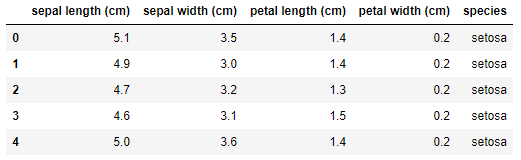iris data.png

我们以iris数据集为例，演示如何使用matplotlib、seaborn、pandas、sklearn进行一维、二维及多维数据可视化，进行探索性数据分析，为后期建模提供一些思路。

二、一维数据可视化

Seaborn是Python基于matplotlib的数据可视化工具。它提供了很多高层封装的函数，帮助数据分析人员快速绘制美观的数据图形，而避免了许多额外的参数配置问题。

用boxplot画出单个特征与species的关系，可以看到不同品种的鸾尾花在petal length单个维度上已经可以较好地划分出来，尤其setosa的petal length跟另外两个品种的petal length差别不要太大好吗，一眼就把你给认出来了。
# look at an individual feature in Seaborn through a boxplot

sns.boxplot(x='species', y='petal length (cm)', data=iris)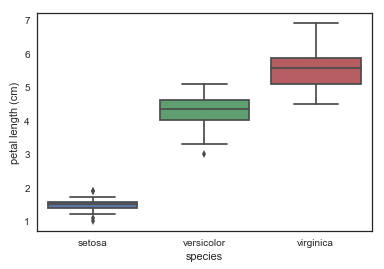box plot

kdeplot核密度图
# kdeplot looking at univariate relations
# creates and visualizes a kernel density estimate of the underlying feature

sns.FacetGrid(iris, hue='species',size=6) \
.map(sns.kdeplot, 'petal length (cm)') \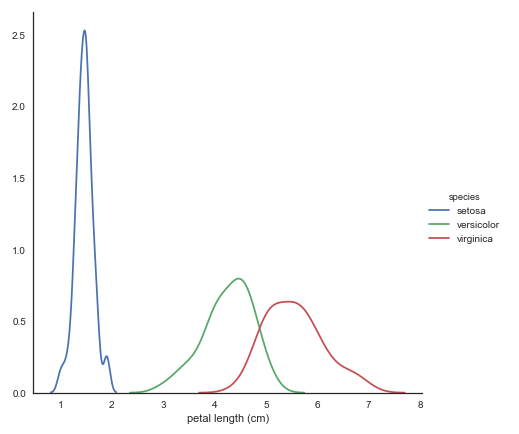kdeplot

violinplot琴形图：结合了箱线图与核密度估计图的特点，它表征了在一个或多个分类变量情况下，连续变量数据的分布并进行了比较，它是一种观察多个数据分布有效方法。
# A violin plot combines the benefits of the boxplot and kdeplot
# Denser regions of the data are fatter, and sparser thiner in a violin plot

sns.violinplot(x='species', y='petal length (cm)', data=iris, size=6)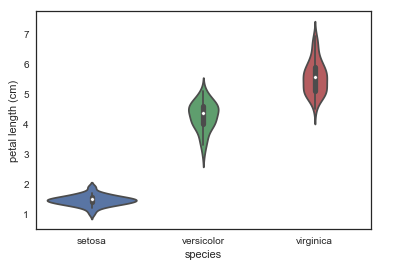violin plot

三、二维数据可视化

散点图：用FacetGrid按照品种标识颜色，便于我们寻找数据间的关系，这里使用了两个特征进行可视化，setosa还是一如既往的好认，virginica跟versicolor还是显得有些难舍难分。
# use seaborn's FacetGrid to color the scatterplot by species

sns.FacetGrid(iris, hue="species", size=5) \
.map(plt.scatter, "sepal length (cm)", "sepal width (cm)") \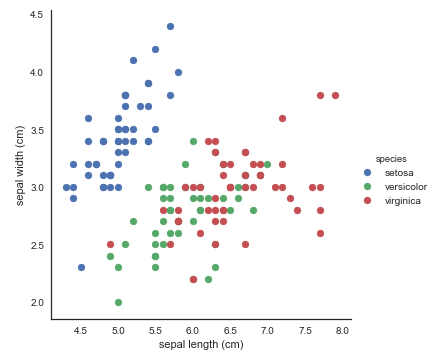scatter plot by species

pairplot：展现特征的两两关系，简直太棒了好吧！
# pairplot shows the bivariate relation between each pair of features
# From the pairplot, we'll see that the Iris-setosa species is separataed from the other two across all feature combinations
# The diagonal elements in a pairplot show the histogram by default
# We can update these elements to show other things, such as a kde

sns.pairplot(iris, hue='species', size=3, diag_kind='kde')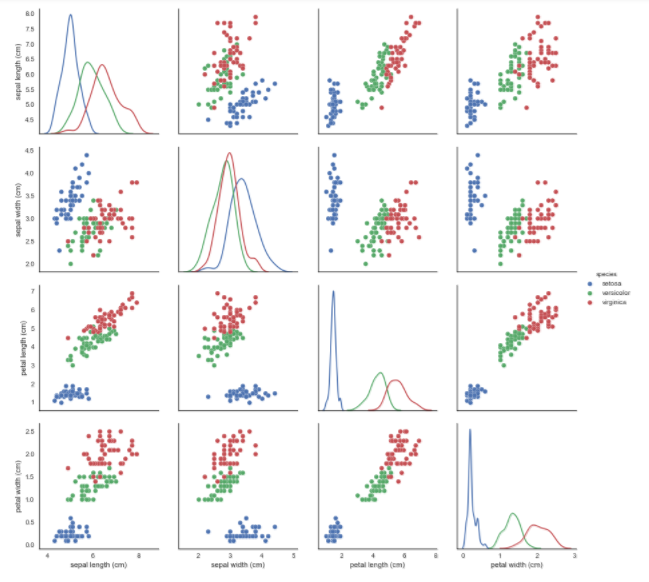pairplot

四、多维数据可视化

这里多维数据可视化不会用到seaborn，主要用到的是pandas、matplotlib和sklearn。

1. Andrews曲线

Andrews曲线将每个样本的属性值转化为傅里叶序列的系数来创建曲线。通过将每一类曲线标成不同颜色可以可视化聚类数据，属于相同类别的样本的曲线通常更加接近并构成了更大的结构。

# Andrews Curves involve using attributes of samples as coefficients for Fourier series and then plotting these

pd.plotting.andrews_curves(iris, 'species')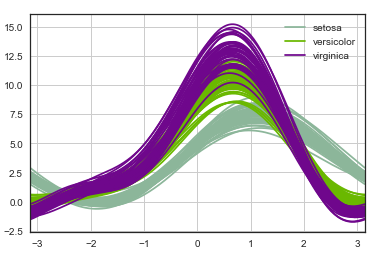andrews curves

2. 平行坐标

平行坐标也是一种多维可视化技术。它可以看到数据中的类别以及从视觉上估计其他的统计量。使用平行坐标时，每个点用线段联接。每个垂直的线代表一个属性。一组联接的线段表示一个数据点。可能是一类的数据点会更加接近。

# Parallel coordinates plots each feature on a separate column & then draws lines connecting the features for each data sample

pd.plotting.parallel_coordinates(iris, 'species')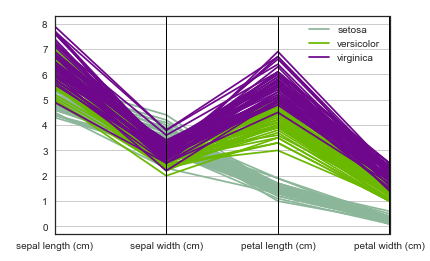parallel coordinates

# radviz  puts each feature as a point on a 2D plane, and then simulates
# having each sample attached to those points through a spring weighted by the relative value for that feature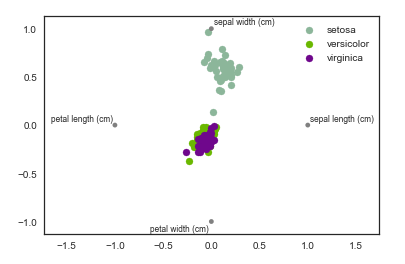4. 因子分析(FactorAnalysis)

因子分析是指研究从变量群中提取共性因子的统计技术。最早由英国心理学家C.E.斯皮尔曼提出。他发现学生的各科成绩之间存在着一定的相关性，一科成绩好的学生，往往其他各科成绩也比较好，从而推想是否存在某些潜在的共性因子，或称某些一般智力条件影响着学生的学习成绩。因子分析可在许多变量中找出隐藏的具有代表性的因子。将相同本质的变量归入一个因子，可减少变量的数目，还可检验变量间关系的假设。

基于高斯潜在变量的一个简单线性模型，假设每一个观察值都是由低维的潜在变量加正态噪音构成。

from sklearn import decomposition

fa = decomposition.FactorAnalysis(n_components=2)

X = fa.fit_transform(iris.iloc[:,:-1].values)

pos=pd.DataFrame()
pos['X'] =X[:, 0]
pos['Y'] =X[:, 1]
pos['species'] = iris['species']

ax = pos[pos['species']=='virginica'].plot(kind='scatter', x='X', y='Y', color='blue', label='virginica')
pos[pos['species']=='setosa'].plot(kind='scatter', x='X', y='Y', color='green', label='setosa', ax=ax)
pos[pos['species']=='versicolor'].plot(kind='scatter', x='X', y='Y', color='red', label='versicolor', ax=ax)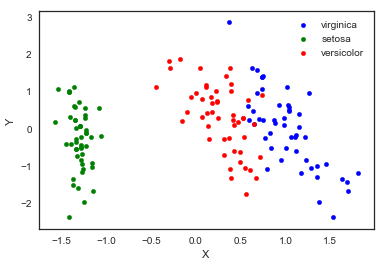fa

5.主成分分析（PCA）

主成分分析是由因子分析进化而来的一种降维的方法，通过正交变换将原始特征转换为线性独立的特征，转换后得到的特征被称为主成分。主成分分析可以将原始维度降维到n个维度，有一个特例情况，就是通过主成分分析将维度降低为2维，这样的话，就可以将多维数据转换为平面中的点，来达到多维数据可视化的目的。

from sklearn import decomposition

pca = decomposition.PCA(n_components=2)

X = pca.fit_transform(iris.iloc[:,:-1].values)

pos=pd.DataFrame()
pos['X'] =X[:, 0]
pos['Y'] =X[:, 1]
pos['species'] = iris['species']

ax = pos[pos['species']=='virginica'].plot(kind='scatter', x='X', y='Y', color='blue', label='virginica')
pos[pos['species']=='setosa'].plot(kind='scatter', x='X', y='Y', color='green', label='setosa', ax=ax)
pos[pos['species']=='versicolor'].plot(kind='scatter', x='X', y='Y', color='red', label='versicolor', ax=ax)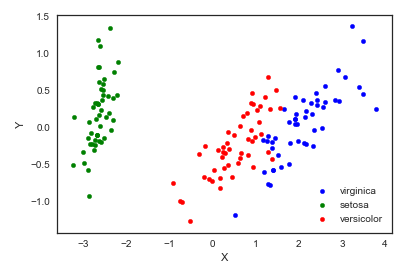pca

需要注意，通过PCA降维实际上是损失了一些信息，我们也可以看一下保留的两个主成分可以解释原始数据的多少。

pca.fit(iris.iloc[:,:-1].values).explained_variance_ratio_


output: array([0.92461621, 0.05301557])
可以看到保留的两个主成分，第一个主成分可以解释原始变异的92.5%，第二个主成分可以解释原始变异的5.3%。也就是说降成两维后仍保留了原始信息的97.8%。

6. 独立成分分析(ICA)

独立成分分析将多源信号拆分成最大可能独立性的子成分，它最初不是用来降维，而是用于拆分重叠的信号。

from sklearn import decomposition

fica = decomposition.FastICA(n_components=2)

X = fica.fit_transform(iris.iloc[:,:-1].values)

pos=pd.DataFrame()
pos['X'] =X[:, 0]
pos['Y'] =X[:, 1]
pos['species'] = iris['species']

ax = pos[pos['species']=='virginica'].plot(kind='scatter', x='X', y='Y', color='blue', label='virginica')
pos[pos['species']=='setosa'].plot(kind='scatter', x='X', y='Y', color='green', label='setosa', ax=ax)
pos[pos['species']=='versicolor'].plot(kind='scatter', x='X', y='Y', color='red', label='versicolor', ax=ax)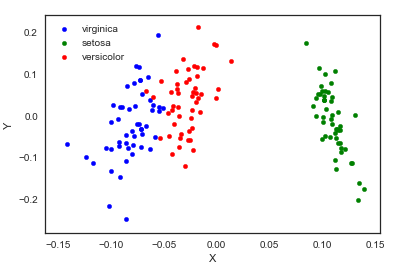ica

7. 多维度量尺（Multi-dimensional scaling, MDS）

多维量表试图寻找原始高维空间数据的距离的良好低维表征。简单来说，多维度量尺被用于数据的相似性，它试图用几何空间中的距离来建模数据的相似性，直白来说就是用二维空间中的距离来表示高维空间的关系。数据可以是物体之间的相似度、分子之间的交互频率或国家间交易指数。这一点与前面的方法不同，前面的方法的输入都是原始数据，而在多维度量尺的例子中，输入是基于欧式距离的距离矩阵。多维度量尺算法是一个不断迭代的过程，因此，需要使用max_iter来指定最大迭代次数，同时计算的耗时也是上面算法中最大的一个。

from sklearn import manifold

from sklearn.metrics import euclidean_distances

similarities = euclidean_distances(iris.iloc[:,:-1].values)
mds = manifold.MDS(n_components=2, max_iter=3000, eps=1e-9, dissimilarity="precomputed", n_jobs=1)
X = mds.fit(similarities).embedding_

pos=pd.DataFrame(X, columns=['X', 'Y'])
pos['species'] = iris['species']

ax = pos[pos['species']=='virginica'].plot(kind='scatter', x='X', y='Y', color='blue', label='virginica')
pos[pos['species']=='setosa'].plot(kind='scatter', x='X', y='Y', color='green', label='setosa', ax=ax)
pos[pos['species']=='versicolor'].plot(kind='scatter', x='X', y='Y', color='red', label='versicolor', ax=ax)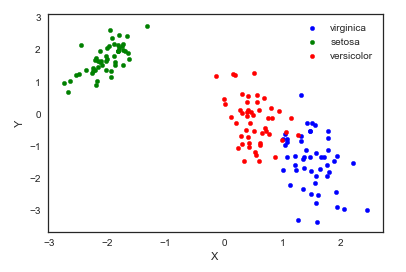mds

8. TSNE（t-distributed Stochastic Neighbor Embedding）

t-SNE(t分布随机邻域嵌入)是一种用于探索高维数据的非线性降维算法。通过基于具有多个特征的数据点的相似性识别观察到的簇来在数据中找到模式，将多维数据映射到适合于人类观察的两个或多个维度。本质上是一种降维和可视化技术。使用该算法的最佳方法是将其用于探索性数据分析。

from sklearn.manifold import TSNE

iris_embedded = TSNE(n_components=2).fit_transform(iris.iloc[:,:-1])

pos = pd.DataFrame(iris_embedded, columns=['X','Y'])
pos['species'] = iris['species']

ax = pos[pos['species']=='virginica'].plot(kind='scatter', x='X', y='Y', color='blue', label='virgnica')
pos[pos['species']=='setosa'].plot(kind='scatter', x='X', y='Y', color='green', label='setosa', ax=ax)
pos[pos['species']=='versicolor'].plot(kind='scatter', x='X', y='Y', color='red', label='versicolor', ax=ax)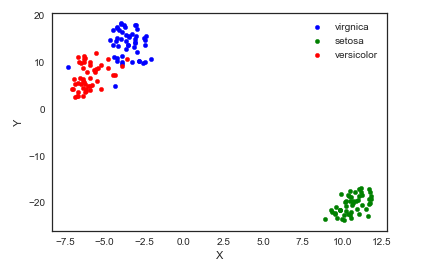TSNE

嗯，我觉得TSNE的结果最可爱了⁄(⁄ ⁄•⁄ω⁄•⁄ ⁄)⁄

五、参考资料

Python Data Visualizations
多维数据可视化
比PCA降维更高级——（R/Python）t-SNE聚类算法实践指南

展开全文• if(count($arr) == count($arr,1)){ echo '一维数组';}else{ echo '多维数组';} 转载于:https://www.cnblogs.com/two-bees/p/10794529.html
if(count($arr) == count($arr,1)){    echo '一维数组';}else{    echo '多维数组';}

转载于:https://www.cnblogs.com/two-bees/p/10794529.html
展开全文• 转自：http://hi.baidu.com/%CD%EE%CE%F7%D1%A7%D4%BA%D6%DC%D3%EE/blog/item/4a154c093116e6a92fddd445.html ...一、一维插值  插值运算是根据数据的分布规律，找到一个函数表达式可以连接已知的各点，并用此函
• 1、numpy创建一维数组 [ 1] 通过列表生成数组 import numpy as np data1=[5,7,9,20]#列表类型 list arr1=np.array(data1) #ndarray数组类型 numpy.ndarray print(data1) print(type(data1)) #type输出数据类型 #...python numpy 经验分享
• 这个问题来源于一个朋友曾经问过我的问题，当时是一个二维数组变成一维数组。后面我想整理一下，整理一个多维，并且是不定维的数组。 一、二维数组变成一维数组 1、遍历数组，将元素一个个放入新数组 结果： ...js es6 数组降维
• 数组与指针之动态分配一维多维数组 1.函数malloc，calloc，realloc功能介绍 2.分配动态数组：首先分配一个指针数组，然后把每个指针初始化为动态分配的“列” 3.删减和扩展动态数组：calloc，realloc函数 指针 malloc 扩展
• # 一维正态分布 # 下面三种方式是等效的 mu = 3 sigma = 0.1 np.random.seed(0) s = np.random.normal(mu, sigma, sampleNo ) plt.subplot(141) plt.hist(s, 30, normed=True) np.random.seed(0) s = sigma * np....
• Python将二维数组/多维数组转换为一维方法1:flatten方法2:reshape+concatenate方法3:sum()方法4:列表推导式方法5:operator方法6:itertools 方法1:flatten import numpy as np mulArrays = [[1,2,3],[4,5,6],[7,8,9]]...
• import java.io.*; import java.util.Arrays; //必须引入java的数组类才可以使用Arrays.toString、Arrays.deepToString这俩函数打印数组 class test {  public static void main ... /*一维数组打印*/  i...java 打印函数
• 数组用于同种数据类型的存储，常规的数组选取的是块连续内存空间来存储同种类型的数据。 1、 静态数组静态数组是在声明时已经确定子数组大小的数组，即数组元素的个数固定不变。在编译期间在栈中分配好内存的...数组与指针的关系 指针
• 来源是朋友问我的一个问题，多维数组的扁平化以及一维数组逆向还原成原始数组。 多维数组的扁平化不必多说，数组实例的flat方法就行。低版本浏览器的话也可以写递归，参考我去年的一篇文章（注：当时写的有点粗糙，......